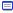naoya_t@topcoder2009-05-20

vectorで多次元配列を作る場合の初期化

| 02:19 |Multidimensional arrays are very important. The simplest way to create the two-dimensional array via vector is to create a vector of vectors.

vector< vector<int> > Matrix;

It should be clear to you now how to create the two-dimensional vector of given size:

int N, M;
// ...
vector< vector<int> > Matrix(N, vector<int>(M, -1));

Here we create a matrix of size N*M and fill it with -1.

Power up C++ with the Standard Template Library: Part I - Algorithm Tutorials

Abusing C++ Extensions for Fun and Profit

| 02:41 |http://www.topcoder.com/tc?module=Static&d1=features&d2=022006

Compound literals

I often define a small struct that will hold two or three items, such as the end-points and weight of an edge in a graph. For two-item structs there is already the utility pair template, but it has unfriendly field names (first and second compared to, say, target and cost), and it doesn't generalise well to more than two elements (although you can create a pair<pair<R, S>, T> if you really insist).

One advantage of pair that you lose when you do this is the make_pair function that creates pairs on the fly. You could of course define such a function for your class, or give it a constructor, but this can take vital coding time. GCC provides another alternative, which comes from C99: the compound literal. A compound literal is a mix between a cast and an initialiser. To understand what I mean, consider a struct defined as

struct edge
{
int target;
int cost;
};

An example of a compound literal is (edge) { t, c } where t and c are expressions. The values in the braces are used to initialise the values of the struct, in the order in which they are declared. This is useful for instantiating structs on-the-fly to pass to STL methods.

Comparisons

Although the header <algorithm> provides min and max functions, these have some limitations. They have only one template parameter, which specifies the type for both arguments; this sometimes leads to errors when the given arguments are of different types. GCC provides the highly non-standard operators ? (max), which will coerce the arguments to a suitable type.

These operators are most commonly seen in their assignment forms ?=. For example, the following loop computes the minimum value of a function over a range:

int m = INT_MAX;
for (int i = 0; i < N; i++)
m <?= func(i);

これは見たことある

トラックバック - http://topcoder.g.hatena.ne.jp/n4_t/20090520